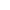# 题解 | #牛牛的单链表求和#

https://www.nowcoder.com/practice/a674715b6b3845aca0d5009bc27380b5

```n = int(input())
lt = list(map(int,input().split()))
class Node:
def __init__(self, val, next=None):
self.val = val
self.next = next
total = lt
for i in lt[1:]:
total += i
print(total)
```